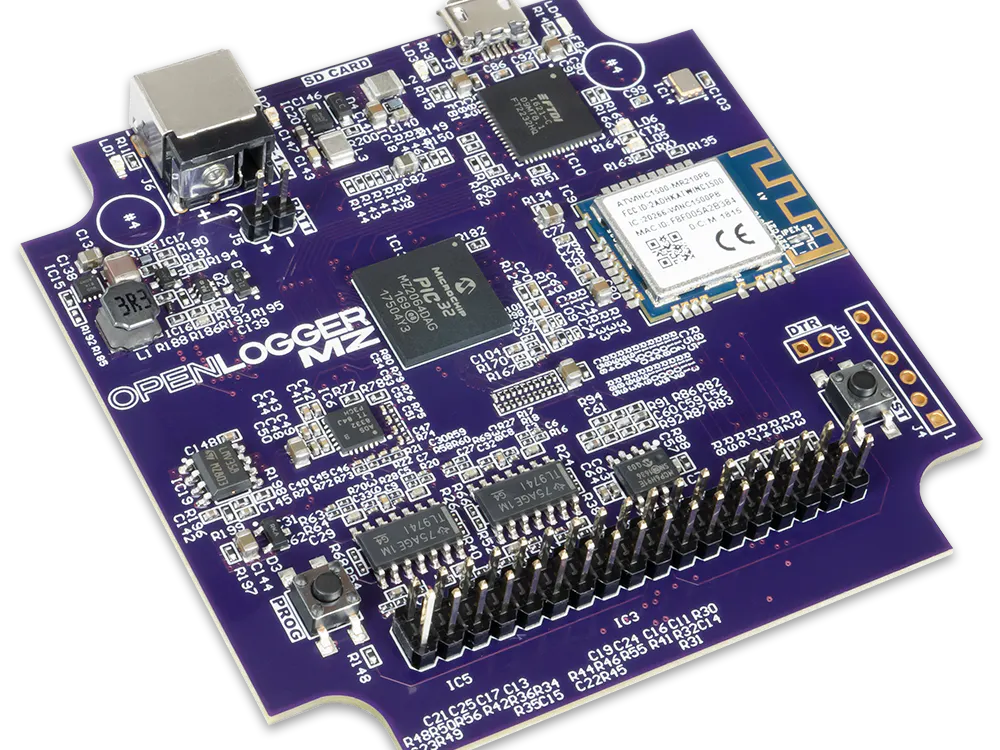# Measuring Power Consumption with the Digilent OpenLogger

How much power does an everyday appliance use? How can we look at and interpret this data?

IntermediateFull instructions provided4 hours1,647## Things used in this project

### Hardware components

 Digilent Openlogger
×1

## Code

### csv_parse.py

Python
Used to calculate RMS current from a CSV file exported from Waveforms Live
```from sys import argv
from math import sqrt
threshold = 0.6
filename = argv#'LoggerData-0.csv'

def first(the_iterable, condition = lambda x: True):
for idx, val in enumerate(the_iterable):
if condition(val):
return idx

with open(filename) as csv_file:
l = s.split('\n')
data = []
for row in l[1:]:
fields = row.split(',')
if fields != ['']:
data.append((
float(fields),
float(fields)
))
print('Number of samples:', len(l[1:]))
trigger_start = first(data, lambda x: x >= threshold or x <= -threshold)
data.reverse()
trigger_end = len(data) - 1 - first(data, lambda x: x >= threshold or x <= -threshold)
data.reverse()
print('buffer clipped:')
print('    start: %d %f' % (trigger_start, data[trigger_start]))
print('    end: %d %f' % (trigger_end, data[trigger_end]))
duration = data[trigger_end] - data[trigger_start]
print('trial duration: %f minutes' % (duration / 60.0))
i_rms = 0.0
for idx in range(trigger_start, trigger_end):
i_rms += data[idx] * data[idx]
i_rms /= trigger_end - trigger_start + 1
i_rms = sqrt(i_rms)
v_rms = 120.0
print('RMS Current: %f Amps' % i_rms)
print('RMS Voltage: %f Voltage' % v_rms)
print('apparent power: %f Watts' % (i_rms * v_rms))
print('Cost per Kilowatt-hour %f' % 0.108) # This is the average for seattle
kwh = (i_rms * v_rms / 1000.0) * (duration / 3600.0)
print('Kilowatt-hours: %f' % kwh)
print('cost of trial: %f' % (kwh * 0.108))

```

## Credits

### Arthur Brown

14 projects • 29 followers
Applications engineer and digital logic geek

### Sam Kristoff

35 projects • 51 followers
R&D Director at NI